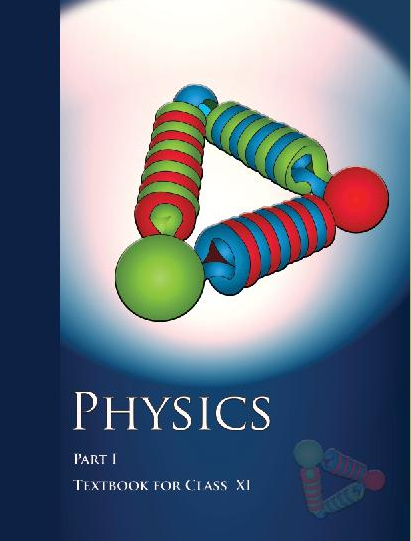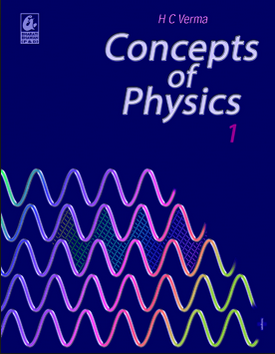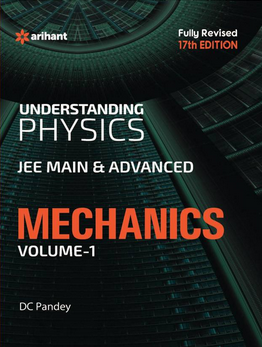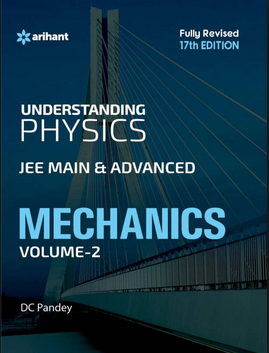# Motion of System Of Particles and Rigid Body (Weightage 4%)   Share

### Topics from Motion of System Of Particles and Rigid Body

• Centre of massof two-particle system (2 concepts)
• Centre of mass of rigid body (16 concepts)
• Basic concepts of rotation (2 concepts)
• Angular momentum and its applications (5 concepts)
• Moment of Inertia introduction (3 concepts)
• Radius of Gyration (1 concepts)
• Values of moments of inertia for simple geometrical objects (9 concepts)
• Parallel and Perpendicular axes theorems and its applications (2 concepts)
• Rigid body rotation (1 concepts)
• Equationof rotational motion (14 concepts)

### Important Books for Motion of System Of Particles and Rigid Body

••••Exams
Articles
Questions